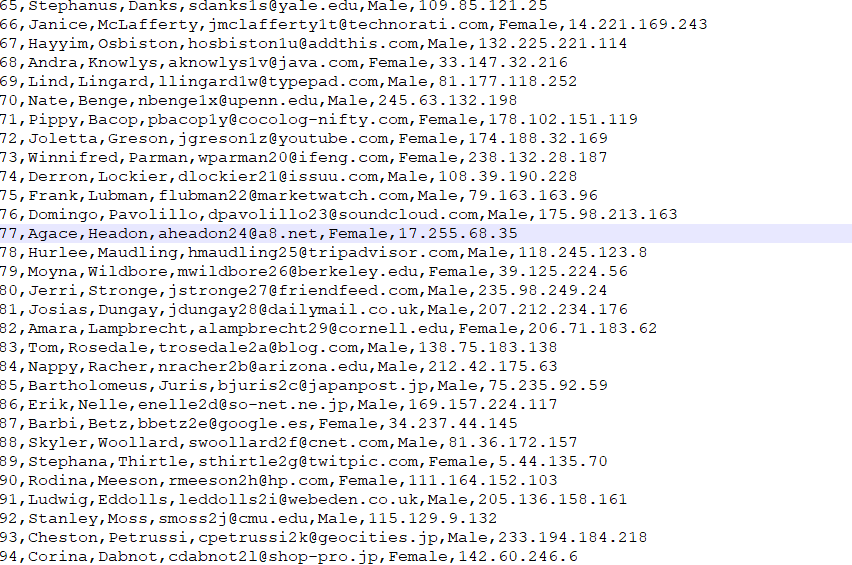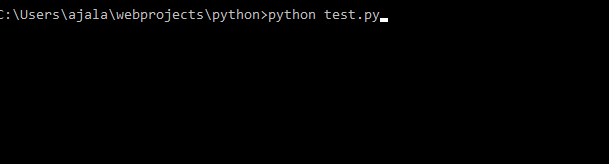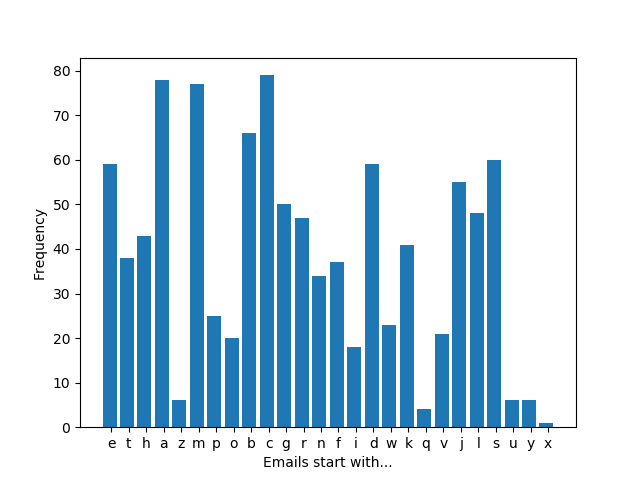## This is so simple, it needs no heading..

08/19/201810:10:49 PM Drinks Coffee as usual…

## Straight to the point:

So this is the CSV data I will be working with ( generated using the website: https://mockaroo.com)MOCK_DATA

## Goal

Create a bar plot showing  the number of emails based on the alphabets they begin with . For instance, how many emails begin with “a” etc… This can be useful for visualizing emails from various email providers (e.g. yahoo, gmail, etc..).

## Code

``````import csv
import matplotlib.pyplot as plt
import numpy as np
import sys
filename = "MOCK_DATA.csv"

def count_email(rows):
dictionary = {}
for row in rows:
email = row
if email not in dictionary:
dictionary[email] = 0
dictionary[email] += 1
return dictionary

def plot_hist(dictionary):
keys = dictionary.keys()
newdata = []
for key in keys:
newdata.append(dictionary[key])
x = np.arange(len(keys))
plt.bar(x, height=newdata)
plt.xticks(x+0.1, keys)
plt.ylabel("Frequency")
plt.show()

data = []

try:
with open(filename) as f:
data = []
data.append(row)
except csv.Error as e:
print("Error while readinf csv file ", e)
sys.exit(-1)
if data:
soln = count_email(data)
print(soln)
if soln:
plot_hist(soln)

``````

### Notes

This assumes your files are all in the same directory ( same level).

## Running the code like this:Produces:Well that was it… if you have suggestions, improvements, questions, do not hesitate to comment down below.

See ya.